### Initialize

using Plots
pyplot()
Plots.PyPlotBackend()

### Lines

A simple line plot of the columns.

plot(Plots.fakedata(50, 5), w = 3)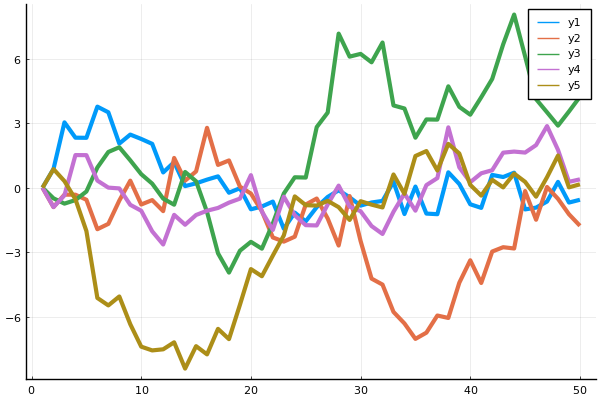### Parametric plots

Plot function pair (x(u), y(u)).

plot(sin, (x->begin
sin(2x)
end), 0, 2π, line = 4, leg = false, fill = (0, :orange))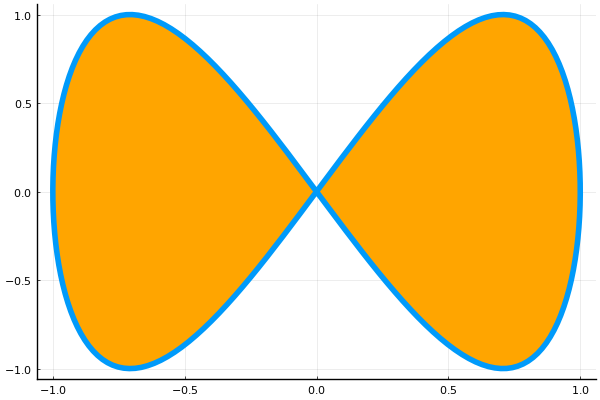### Colors

Access predefined palettes (or build your own with the colorscheme method). Line/marker colors are auto-generated from the plot's palette, unless overridden. Set the z argument to turn on series gradients.

y = rand(100)
plot(0:10:100, rand(11, 4), lab = "lines", w = 3, palette = cgrad(:grays), fill = 0, α = 0.6)
scatter!(y, zcolor = abs.(y .- 0.5), m = (:heat, 0.8, Plots.stroke(1, :green)), ms = 10 * abs.(y .- 0.5) .+ 4, lab = "grad")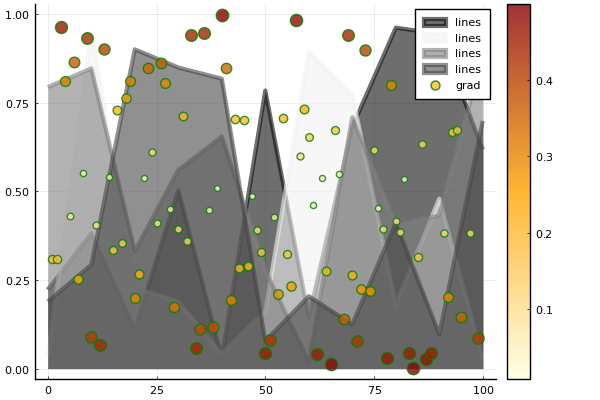### Global

Change the guides/background/limits/ticks. Convenience args xaxis and yaxis allow you to pass a tuple or value which will be mapped to the relevant args automatically. The xaxis below will be replaced with xlabel and xlims args automatically during the preprocessing step. You can also use shorthand functions: title!, xaxis!, yaxis!, xlabel!, ylabel!, xlims!, ylims!, xticks!, yticks!

using Statistics
y = rand(20, 3)
plot(y, xaxis = ("XLABEL", (-5, 30), 0:2:20, :flip), background_color = RGB(0.2, 0.2, 0.2), leg = false)
hline!(mean(y, dims = 1) + rand(1, 3), line = (4, :dash, 0.6, [:lightgreen :green :darkgreen]))
vline!([5, 10])
title!("TITLE")
yaxis!("YLABEL", :log10, minorgrid = true)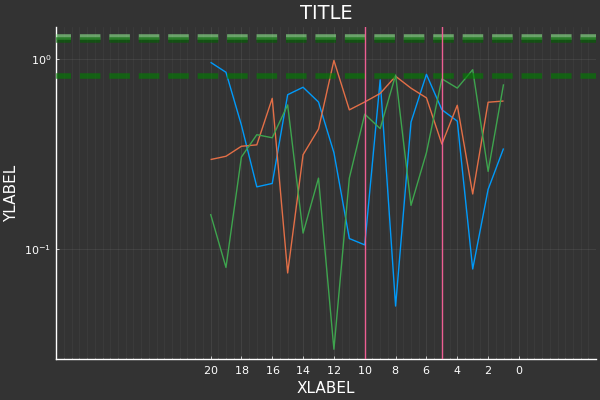### Images

Plot an image. y-axis is set to flipped

import FileIO
plot(img)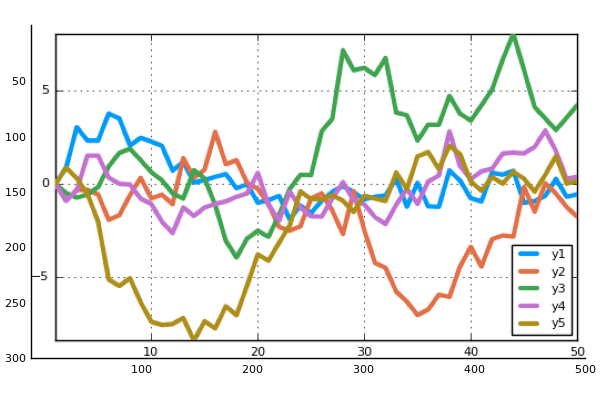### Arguments

Plot multiple series with different numbers of points. Mix arguments that apply to all series (marker/markersize) with arguments unique to each series (colors). Special arguments line, marker, and fill will automatically figure out what arguments to set (for example, we are setting the linestyle, linewidth, and color arguments with line.) Note that we pass a matrix of colors, and this applies the colors to each series.

ys = Vector[rand(10), rand(20)]
plot(ys, color = [:black :orange], line = (:dot, 4), marker = ([:hex :d], 12, 0.8, Plots.stroke(3, :gray)))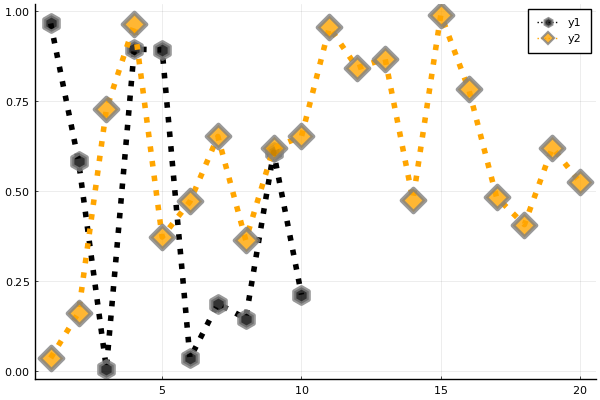### Build plot in pieces

plot(rand(100) / 3, reg = true, fill = (0, :green))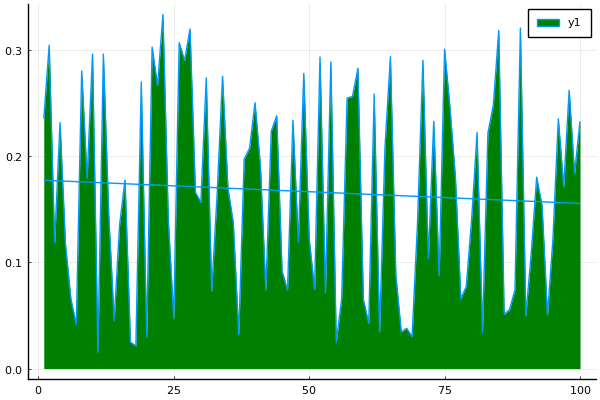scatter!(rand(100), markersize = 6, c = :orange)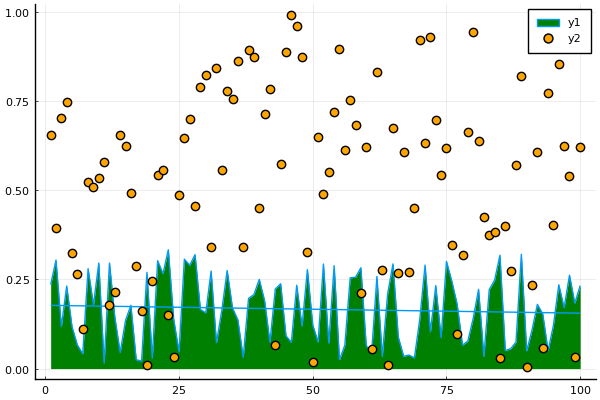### Histogram2D

histogram2d(randn(10000), randn(10000), nbins = 20)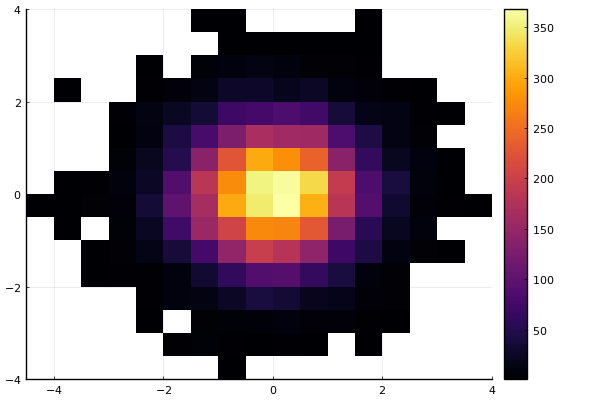### Line types

linetypes = [:path :steppre :steppost :sticks :scatter]
n = length(linetypes)
x = Vector[sort(rand(20)) for i = 1:n]
y = rand(20, n)
plot(x, y, line = (linetypes, 3), lab = map(string, linetypes), ms = 15)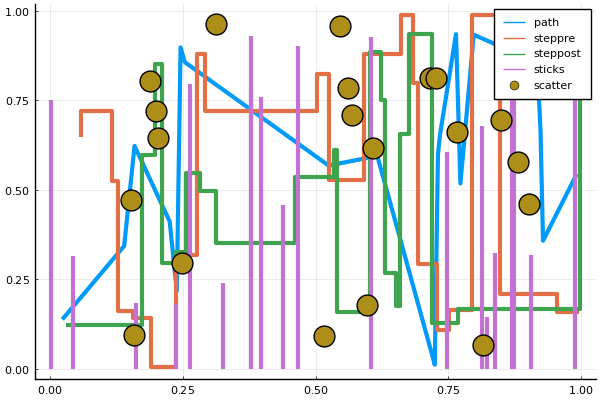### Line styles

styles = filter((s->begin
s in Plots.supported_styles()
end), [:solid, :dash, :dot, :dashdot, :dashdotdot])
styles = reshape(styles, 1, length(styles))
n = length(styles)
y = cumsum(randn(20, n), dims = 1)
plot(y, line = (5, styles), label = map(string, styles), legendtitle = "linestyle")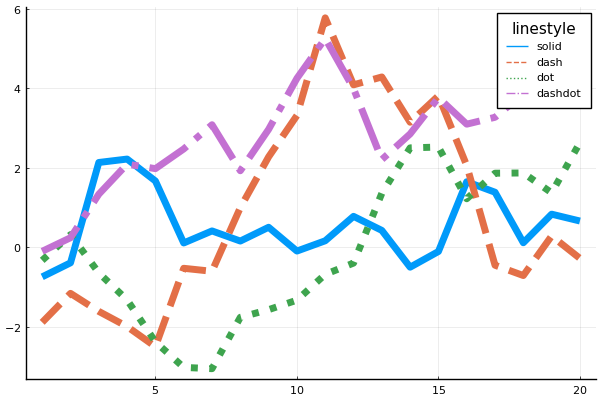### Marker types

markers = filter((m->begin
m in Plots.supported_markers()
end), Plots._shape_keys)
markers = permutedims(markers)
n = length(markers)
x = (range(0, stop = 10, length = n + 2))[2:end - 1]
y = repeat(reshape(reverse(x), 1, :), n, 1)
scatter(x, y, m = markers, markersize = 8, lab = map(string, markers), bg = :linen, xlim = (0, 10), ylim = (0, 10))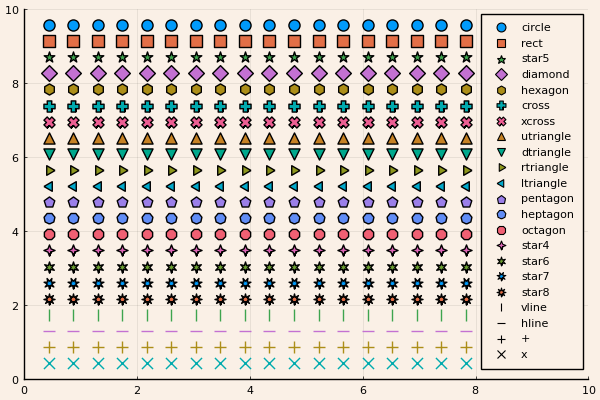### Bar

x is the midpoint of the bar. (todo: allow passing of edges instead of midpoints)

bar(randn(99))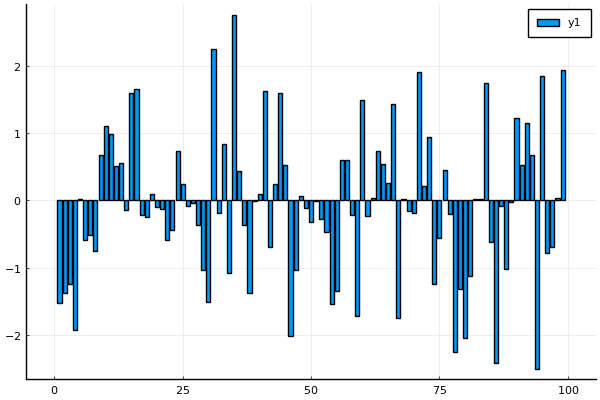### Histogram

histogram(randn(1000), bins = :scott, weights = repeat(1:5, outer = 200))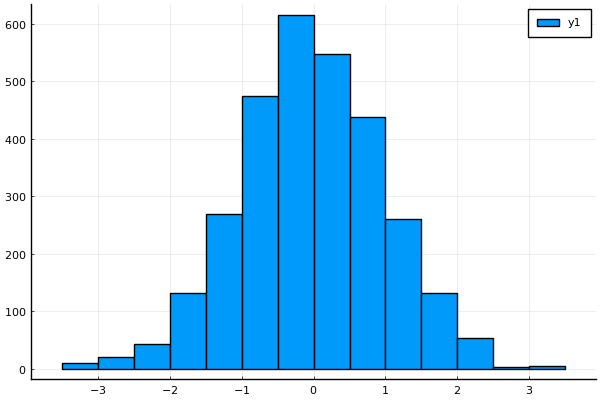### Subplots

Use the layout keyword, and optionally the convenient @layout macro to generate arbitrarily complex subplot layouts.

l = @layout([a{0.1h}; b [c; d e]])
plot(randn(100, 5), layout = l, t = [:line :histogram :scatter :steppre :bar], leg = false, ticks = nothing, border = :none)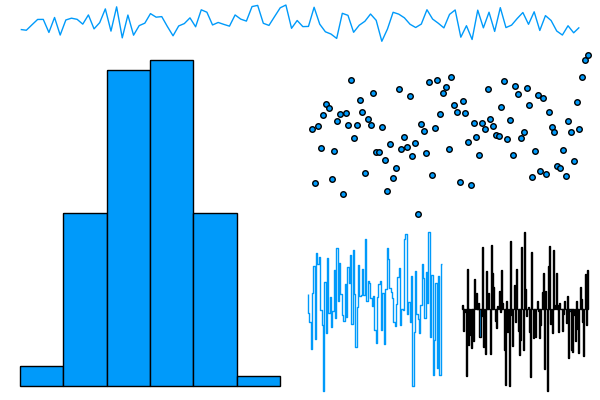plot(Plots.fakedata(100, 10), layout = 4, palette = cgrad.([:grays :blues :heat :lightrainbow]), bg_inside = [:orange :pink :darkblue :black])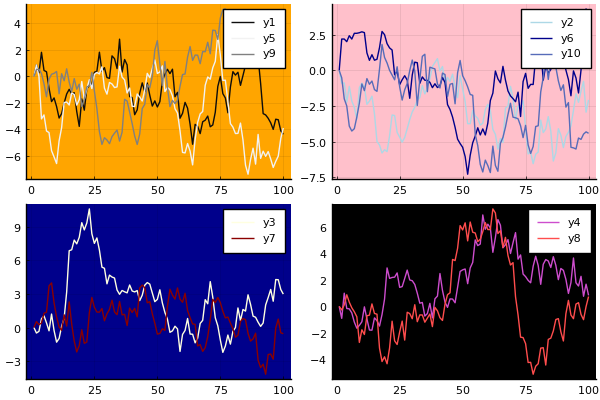using Random
plot!(Plots.fakedata(100, 10))